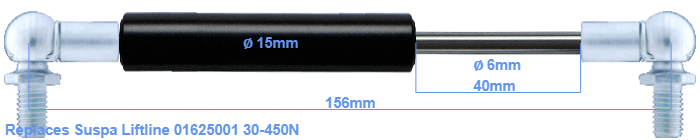# Replacement for Suspa Liftline 01625001 30-450N

\$38.05

Replacement gas spring for the Suspa Liftline 01625001 30-450 Newton. This fits a (possibly already present) ball with a diameter of 10mm. A corresponding ball is included. Brand: Stabilus Industry Line.
 Force Choose an option30 Newton40 Newton50 Newton60 Newton80 Newton100 Newton120 Newton140 Newton150 Newton160 Newton180 Newton200 Newton220 Newton240 Newton250 Newton260 Newton280 Newton300 Newton320 Newton340 Newton350 Newton360 Newton380 Newton400 Newton420 Newton440 Newton450 NewtonClear
This gas spring is also known as 16-1 016 25001, 16-1-72,5-45-A246-B246.The diameter of the cylinder of this gas spring is 15 mm. The diameter of the rod totals 6 mm. The stroke (the retracting part) has a length of 40 mm. In total, the length is 156 millimeter. This is the distance between the centers of the mounting parts. The distance from thread to thread (without mounting parts) is 112 mm. The force of this replacement gas spring is 30-450 Newton. This is not an official Suspa Liftline gas spring, but a so called replacement. This replacement gas spring is a Stabilus Industry Line gas spring. Nevertheless, this gas spring can be used as replacement gas spring, because the dimensions and forces are equal.
Category: